Book preview
Theory  of  everything

The Final Formula

Many physical phenomena can be explained with the fundamental natural constants of speed of light and the
reduced Planck's quantum of action. In the new world model the following context constitutes the base of
each physical event in the universe, and it can therefore be defined as a "final formula".

The final formula:

Speed of light with c=299.792.458 m/s
Reduced Planck constant with:

The reduced Planck quantum of action has been modified by transforming the final formula with:

Planck's quantum of action is determined experimentally, and physical measurements are always associated
with measurement errors. Even the most accurate measurement can not provide a precise value. The
determination of Planck's quantum of action depends on many factors, so with the above reduced formula of
the Planck constant was modified. The absolute deviation from the CODATA value is minimal and amounts:
.

The final formula has the dimension of energy times length and thereby constitutes a universal relationship
between energy content and length scale. It describes the amount of energy contained in the space balls and
here, the quantized length amounts as modified Planck length. The dimension of the final formula with the
general units eV in nuclear physics is:

197, 39208802178700 MeVfm

Despite to the above modified h, the modified value from the next Chapter was used for the elementary
charge e.

The final formula means that the space balls with the diameter of Plancklength contain a certain
amount of energy. The interaction between the space balls is done with the time factor contained
in the speed of light and the smallest action h.

The constant speed of light is used as the time factor in the pulse transmission and therefore, also the
quantized action h is constant. When asked which of the two existed at first (Hen-egg problem), we can say
with certainty that the time factor which is measured from the speed of light is given, and that h is directly
dependent. In the Chapter "derivation of the speed of light," we will continue to discuss the phenomenon of
the time factor.

The attraction in the space balls in the form of energy with the number square root of 10 and the universal
time factor with the speed of light, are the only basic elements with which the universe is built. The modified
Planck quantum of action as the smallest effect also plays a big role, but this quantity directly depends on the
speed of light and therefore can be derived from it.

With the "final formula" it is possible to make a clear and unambiguous description of the phenomena
observed in nature. The simple formula also allows predictions about new phenomena.

The final formula is harmless and any concerns that zealous scientists could use it to play "God" with it and
turn the world upside down, are unfounded. The left side of the formula contains natural constants that have
long been known for a long time and are used daily in nuclear physics. My discovery relates to the right side
of the formula, and it explains to what the fundamental constants of nature, the speed of light and the Planck
constant can be attributed to.

According to the new world model, space and time is quantized in the universe. In addition to the elementary
magnitudes such as the Planck mass, the Planck charge etc., also some fundamental constants of nature, and
even the speed of light can be derived with the final formula. We also will gain new insights on the nature of
time and the three-dimensionality of space.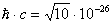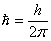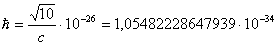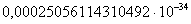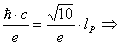Copyright © 2012 Halit Eroglu - Theory of everything  -       - Imprint - Contact - Sitemap